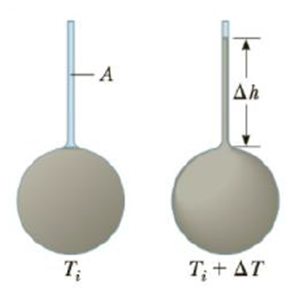Chapter 10, Problem 61AP

Chapter
Section
Textbook Problem

A liquid with a coefficient of volume expansion of β just fills a spherical flask of volume V0 at temperature Ti (Fig. P10.61). The flask is made of a material that has a coefficient of linear expansion of α. The liquid is free to expand into a capillary of cross-sectional area A at the top. (a) Show that if the temperature increases by ΔT, the liquid rises in the capillary by the amount Δh = (V0/A)(β − 3α)ΔT. (b) For a typical system, such as a mercury thermometer, why is it a approximation to neglect the expansion of the flask?Figure P10.61

(a)

To determine
The rise in the liquid ( Δh ).

Explanation

Given info: The co-efficient of volume expansion of the liquid is β . The volume of the spherical flask is V0 . The temperature of the flask is Ti . The co-efficient of linear expansion of the flask is α . The cross sectional area of the capillary is A. The temperature increases by ΔT .

Formula for the overflow volume is,

Vof=AΔh (I)

Overflow volume is also given by,

Vof=ΔVlΔVf (II)

• ΔVl is the change in volume of the liquid.
• ΔVf is the change in volume of the flask.

Formula to calculate ΔVl is,

ΔVl=βVoΔT (III)

Formula to calculate ΔVf is,

ΔVf=3αVoΔT (IV)

Substitute Equations (III) and (IV) in (II)

(b)

To determine
Why the expansion of the flask is neglected for mercury.

Still sussing out bartleby?

Check out a sample textbook solution.

See a sample solution

The Solution to Your Study Problems

Bartleby provides explanations to thousands of textbook problems written by our experts, many with advanced degrees!

Get Started

What is hereditarianism, and what is the invalid assumption it makes?

Human Heredity: Principles and Issues (MindTap Course List)

8.35 What is an instantancous dipole?

Chemistry for Engineering Students

Cell junctions called__________ connect the cytoplasm of plant cells.

Biology: The Unity and Diversity of Life (MindTap Course List)